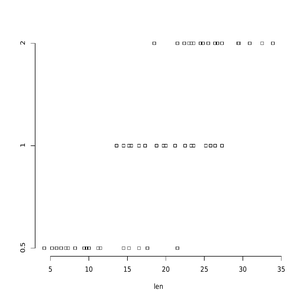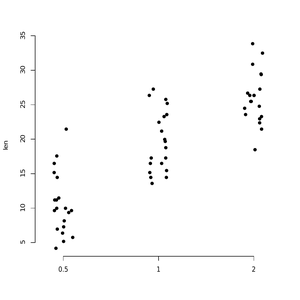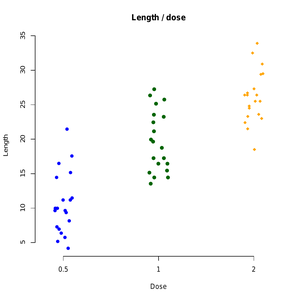# Create One Dimensional Scatterplots in R Programming – stripchart() Function

Strip Chart is defined as one dimensional scatter plots or dot plots which is used as an alternative to box plot when sample sizes are small. It is created using the `stripchart()` function in R Language.

Syntax:
stripchart(x, data = NULL method, jitter )

Parameters:
x: value of data from which the plots are to be produced.
data: a data.frame from which the variables in x value should be taken.
Method: the method is basically used to separate coincident points.
jitter: when method = “jitter” is used, jitter will produce the amount of jittering applied.

Approach:

• This example make use of ToothGrowth data base
• stripchart() Function is implemented using ToothGrowth data base.

Dataset:

 `ToothGrowth\$dose <- ``as.factor``(ToothGrowth\$dose) ` ` `  `# Print the first 7 rows ` `head``(ToothGrowth, 7) `

Output:

```   len supp dose
1  4.2   VC  0.5
2 11.5   VC  0.5
3  7.3   VC  0.5
4  5.8   VC  0.5
5  6.4   VC  0.5
6 10.0   VC  0.5
7 11.2   VC  0.5```

Above code will print data set

Example 1: Simple Stripchart

 `# Plot len by dose ` `stripchart``(len ~ dose, data = ToothGrowth, ` `         ``pch = 22, frame = ``FALSE``) `

Output:Example 2: Plot vertical stripchart

 `# Vertical plot using method = "jitter" ` `stripchart``(len ~ dose, data = ToothGrowth, ` `         ``pch = 16, frame = ``FALSE``, vertical = ``TRUE``, ` `         ``method = ``"jitter"``) `

Output:Example 3: Change in point shapes (pch) and colors by groups

 `# Change point shapes (pch) and colors by groups ` `# add main title and axis labels ` `stripchart``(len ~ dose, data = ToothGrowth, ` `         ``frame = ``FALSE``, vertical = ``TRUE``, ` `         ``method = ``"jitter"``, pch = ``c``(16, 19, 18), ` `         ``col = ``c``(``"blue "``, ``"darkgreen"``, ``"orange"``), ` `         ``main = ``"Length / dose"``, xlab = ``"Dose"``, ylab = ``"Length"``) `

Output:Above example will add the main title and axis labels and print colored stripcharts.

My Personal Notes arrow_drop_upCheck out this Author's contributed articles.

If you like GeeksforGeeks and would like to contribute, you can also write an article using contribute.geeksforgeeks.org or mail your article to contribute@geeksforgeeks.org. See your article appearing on the GeeksforGeeks main page and help other Geeks.

Please Improve this article if you find anything incorrect by clicking on the "Improve Article" button below.

Article Tags :

Be the First to upvote.

Please write to us at contribute@geeksforgeeks.org to report any issue with the above content.